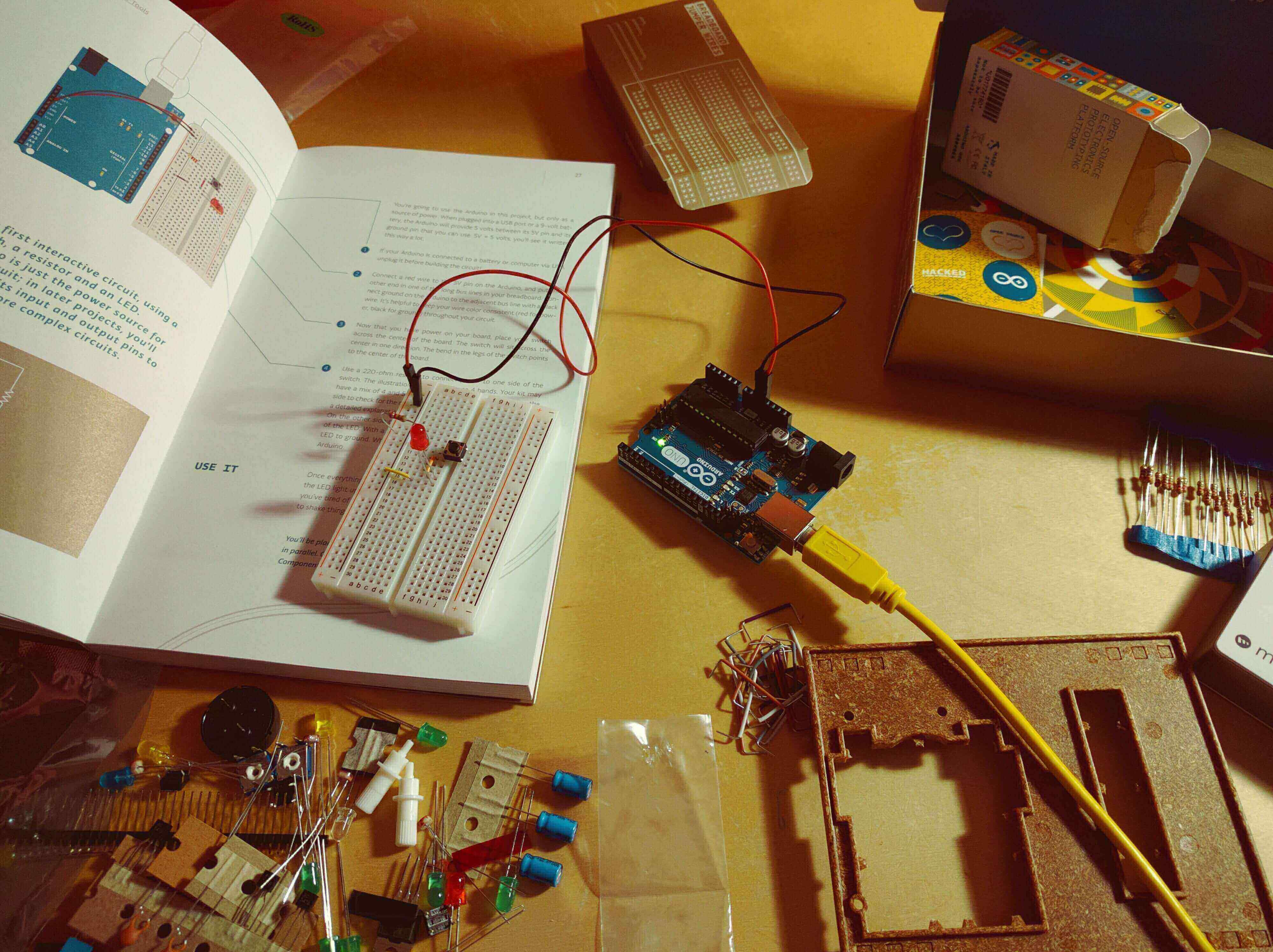# Signal and System ( EC-402 )

( EC- 4th Sem )### Course Outcomes

After Completion of this Course students can:

• Understand concepts of Signals and Systems,definition of system and their classification.
• Understand concepts of Linear Time- Invariant Systems.
• Understand concepts of z-Transform.
• Understand concepts of Fourier analysis of discrete time signals.
• Understand concepts of State Space.

### Course Content

• Definition of signal, Classification of Signal and representation: Continuous time and discrete time, even/odd, periodic/aperiodic, random/deterministic, energy/power, one/multidimensional, some standard signals, , Basic Operations on Signals for CT/DT signal, transformation of independent & dependent variables,CT/DT, linear/non-linear, variant/non-variant, causal and non-causal system state/dynamic system, interconnection of systems. System properties: linearity: additivity and homogeneity, shift-invariance, causality, stability, realizability
• Introduction, Impulse Response Representation for LTI Systems, Convolution, Properties of the Impulse Response Representation for LTI Systems, Difference Equation for LTI Systems, Block Diagram Representations(direct form-I, direct formII, Transpose, cascade and parallel). Impulse response of DT-LTI system and its properties.
• Introduction, ROC of finite duration sequence, ROC of infinite duration sequence, Relation between Discrete time Fourier Transform and z-transform, properties of the ROC, Properties of z-transform, Inverse z-Transform, Analysis of discrete time LTI system using zTransform, Unilateral z-Transform.
• Introduction, Properties and application of discrete time Fourier series, Representation of Aperiodic signals, Fourier transform and its properties, Convergence of discrete time Fourier transform, Fourier Transform for periodic signals, Applications of DTFT.
• State-space analysis and multi-input, multi-output representation. The state-transition matrix and its role. The Sampling Theorem and its implications- Spectra of sampled signals. Reconstruction

### Book References

• Simon Haykin, “Signals and Systems”, John Wiley
• Simon Haykin, “Analog and Digital Communications”, John Willey.
• Bruce Carlson, “Signals and Systems”, TMH.
• Oppenheim & Wilsky, “Signals & Systems”, PHI
• Taub and Schilling "Principles of communication signals", 2nd ed. New York: McgrawHill, 1986.

### List of Experiments

• Introduction to MATLAB Tool.
• To implement delta function, unit step function, ramp function and parabolic function for continuous-time.
• To implement delta function, unit step function, ramp function and parabolic function for discrete-time.
• To implement rectangular function, triangular function, sinc function and signum function for continuous-time.
• To implement rectangular function, triangular function, sinc function and signum function for discrete-time.
• To explore the communication of even and odd symmetries in a signal with algebraic operations.
• To explore the effect of transformation of signal parameters (amplitude-scaling, timescaling & shifting).
• To explore the time variance and time invariance property of a given system.
• To explore causality and non-causality property of a system.
• To demonstrate the convolution of two continuous-time signals.

• To perform various arithmetic operatin using scilab.
• Write scilab code to show marks of 10 students in bargraph.
• To draw discrete and continous unit step function on scilab.
• Scilab code for unit and ramp continous and discrete signal and exponential continous and discrete signal.
• To generate addition and subtraction of two given signal in discontinous domain.
• To generate signal shifting folding, additon, subtractions.

• Exp -1
• Exp -2
• Exp -3
• Exp -4
• Exp -5
• Exp -6

### Cousre Notes

For "Course Notes" you can refer our Notes Section for 2ndYear( Click Here )

• TAGS:

•College Academy
Author- Himanshu
( Core Member )